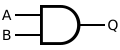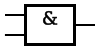# AND gate facts for kids

Kids Encyclopedia Facts
 INPUT OUTPUT A B A AND B 0 0 0 0 1 0 1 0 0 1 1 1

The AND gate is a logic gate that outputs 1 (true) only when both of its inputs are 1 (true). Since 0 is false, AND outputs the product (result of multiplication) of its inputs. That means that if either or both of its inputs are 0, the output will be 0 (zero multiplied by any real number is zero).

## Symbols

There are three symbols for the AND gate:MIL/ANSI Symbol IEC Symbol DIN Symbol

## Images for kidsAND gate Facts for Kids. Kiddle Encyclopedia.